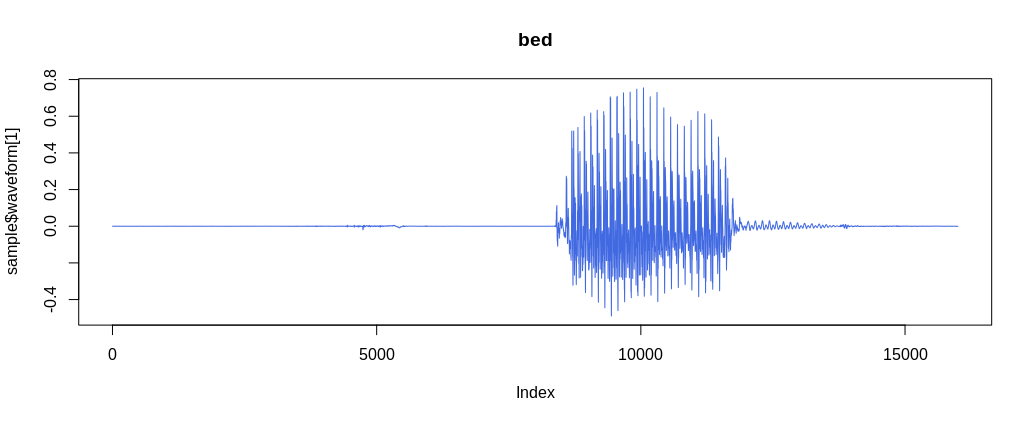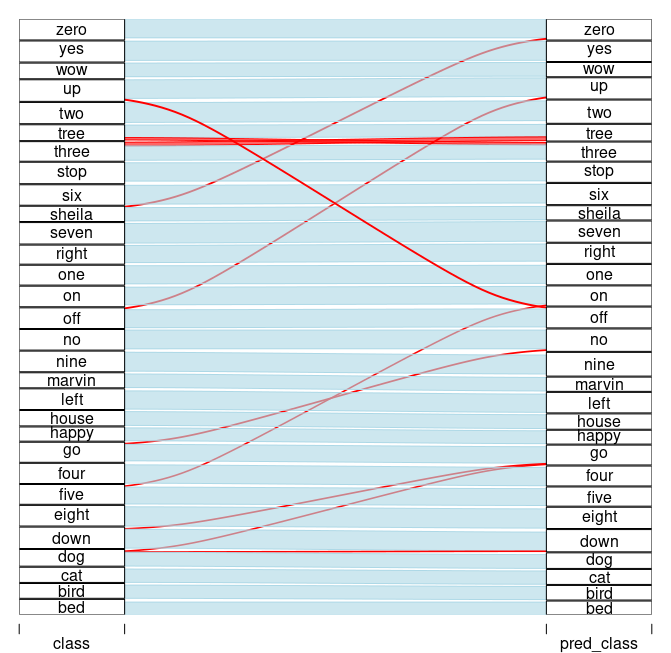# Simple audio classification with torch

This article translates Daniel Falbel’s post on “Simple Audio Classification” from TensorFlow/Keras to torch/torchaudio.

Athos Damiani (Curso-R)https://curso-r.com/
02-04-2021

This article translates Daniel Falbel’s ‘Simple Audio Classification’ article from `tensorflow/keras` to `torch/torchaudio`. The main goal is to introduce torchaudio and illustrate its contributions to the `torch` ecosystem. Here, we focus on a popular dataset, the audio loader and the spectrogram transformer. An interesting side product is the parallel between torch and tensorflow, showing sometimes the differences, sometimes the similarities between them.

``````library(torch)
library(torchaudio)``````

torchaudio has the `speechcommand_dataset` built in. It filters out background_noise by default and lets us choose between versions `v0.01` and `v0.02`.

``````# set an existing folder here to cache the dataset
DATASETS_PATH <- "~/datasets/"

df <- speechcommand_dataset(
root = DATASETS_PATH,
url = "speech_commands_v0.01",
)

# expect folder: _background_noise_
df\$EXCEPT_FOLDER
#  "_background_noise_"

# number of audio files
length(df)
#  64721

# a sample
sample <- df

sample\$waveform[, 1:10]``````
``````torch_tensor
0.0001 *
0.9155  0.3052  1.8311  1.8311 -0.3052  0.3052  2.4414  0.9155 -0.9155 -0.6104
[ CPUFloatType{1,10} ]``````
``````sample\$sample_rate
# 16000
sample\$label
# bed

plot(sample\$waveform, type = "l", col = "royalblue", main = sample\$label)``````Figure 1: A sample waveform for a ‘bed’.

## Classes

``df\$classes``
``````  "bed"    "bird"   "cat"    "dog"    "down"   "eight"  "five"
 "four"   "go"     "happy"  "house"  "left"   "marvin" "nine"
 "no"     "off"    "on"     "one"    "right"  "seven"  "sheila"
 "six"    "stop"   "three"  "tree"   "two"    "up"     "wow"
 "yes"    "zero"  ``````

`torch::dataloader` has the same task as `data_generator` defined in the original article. It is responsible for preparing batches - including shuffling, padding, one-hot encoding, etc. - and for taking care of parallelism / device I/O orchestration.

In torch we do this by passing the train/test subset to `torch::dataloader` and encapsulating all the batch setup logic inside a `collate_fn()` function.

``````set.seed(6)
id_train <- sample(length(df), size = 0.7*length(df))
id_test <- setdiff(seq_len(length(df)), id_train)
# subsets

train_subset <- torch::dataset_subset(df, id_train)
test_subset <- torch::dataset_subset(df, id_test)``````

At this point, `dataloader(train_subset)` would not work because the samples are not padded. So we need to build our own `collate_fn()` with the padding strategy.

I suggest using the following approach when implementing the `collate_fn()`:

1. begin with `collate_fn <- function(batch) browser()`.
2. instantiate `dataloader` with the `collate_fn()`
3. create an environment by calling `enumerate(dataloader)` so you can ask to retrieve a batch from dataloader.
4. run `environment[][]`. Now you should be sent inside collate_fn() with access to `batch` input object.
5. build the logic.
``````collate_fn <- function(batch) {
browser()
}

train_subset,
batch_size = 32,
shuffle = TRUE,
collate_fn = collate_fn
)

ds_train_env <- enumerate(ds_train)
ds_train_env[][]``````

The final `collate_fn()` pads the waveform to length 16001 and then stacks everything up together. At this point there are no spectrograms yet. We going to make spectrogram transformation a part of model architecture.

``````pad_sequence <- function(batch) {
# Make all tensors in a batch the same length by padding with zeros
batch <- sapply(batch, function(x) (x\$t()))
return(batch\$permute(c(1, 3, 2)))
}

# Final collate_fn
collate_fn <- function(batch) {
# Input structure:
# list of 32 lists: list(waveform, sample_rate, label, speaker_id, utterance_number)
# Transpose it
batch <- purrr::transpose(batch)
tensors <- batch\$waveform
targets <- batch\$label_index

# Group the list of tensors into a batched tensor

# target encoding
targets <- torch::torch_stack(targets)

list(tensors = tensors, targets = targets) # (64, 1, 16001)
}``````

Batch structure is:

• batch[]: waveforms - `tensor` with dimension (32, 1, 16001)
• batch[]: targets - `tensor` with dimension (32, 1)

Also, torchaudio comes with 3 loaders, `av_loader`, `tuner_loader`, and `audiofile_loader`- more to come. `set_audio_backend()` is used to set one of them as the audio loader. Their performances differ based on audio format (mp3 or wav). There is no perfect world yet: `tuner_loader` is best for mp3, `audiofile_loader` is best for wav, but neither of them has the option of partially loading a sample from an audio file without bringing all the data into memory first.

For a given audio backend we need pass it to each worker through `worker_init_fn()` argument.

``````ds_train <- dataloader(
train_subset,
batch_size = 128,
shuffle = TRUE,
collate_fn = collate_fn,
num_workers = 16,
)

test_subset,
batch_size = 64,
shuffle = FALSE,
collate_fn = collate_fn,
num_workers = 8,
)``````

## Model definition

Instead of `keras::keras_model_sequential()`, we are going to define a `torch::nn_module()`. As referenced by the original article, the model is based on this architecture for MNIST from this tutorial, and I’ll call it ‘DanielNN’.

``````dan_nn <- torch::nn_module(
"DanielNN",

initialize = function(
window_size_ms = 30,
window_stride_ms = 10
) {

# spectrogram spec
window_size <- as.integer(16000*window_size_ms/1000)
stride <- as.integer(16000*window_stride_ms/1000)
fft_size <- as.integer(2^trunc(log(window_size, 2) + 1))
n_chunks <- length(seq(0, 16000, stride))

self\$spectrogram <- torchaudio::transform_spectrogram(
n_fft = fft_size,
win_length = window_size,
hop_length = stride,
normalized = TRUE,
power = 2
)

# convs 2D
self\$conv1 <- torch::nn_conv2d(in_channels = 1, out_channels = 32, kernel_size = c(3,3))
self\$conv2 <- torch::nn_conv2d(in_channels = 32, out_channels = 64, kernel_size = c(3,3))
self\$conv3 <- torch::nn_conv2d(in_channels = 64, out_channels = 128, kernel_size = c(3,3))
self\$conv4 <- torch::nn_conv2d(in_channels = 128, out_channels = 256, kernel_size = c(3,3))

# denses
self\$dense1 <- torch::nn_linear(in_features = 14336, out_features = 128)
self\$dense2 <- torch::nn_linear(in_features = 128, out_features = 30)
},

forward = function(x) {
x %>% # (64, 1, 16001)
self\$spectrogram() %>% # (64, 1, 257, 101)
torch::torch_log() %>%
self\$conv1() %>%
torch::nnf_relu() %>%
torch::nnf_max_pool2d(kernel_size = c(2,2)) %>%

self\$conv2() %>%
torch::nnf_relu() %>%
torch::nnf_max_pool2d(kernel_size = c(2,2)) %>%

self\$conv3() %>%
torch::nnf_relu() %>%
torch::nnf_max_pool2d(kernel_size = c(2,2)) %>%

self\$conv4() %>%
torch::nnf_relu() %>%
torch::nnf_max_pool2d(kernel_size = c(2,2)) %>%

torch::nnf_dropout(p = 0.25) %>%
torch::torch_flatten(start_dim = 2) %>%

self\$dense1() %>%
torch::nnf_relu() %>%
torch::nnf_dropout(p = 0.5) %>%
self\$dense2()
}
)

model <- dan_nn()

device <- torch::torch_device(if(torch::cuda_is_available()) "cuda" else "cpu")
model\$to(device = device)

print(model)``````
``````An `nn_module` containing 2,226,846 parameters.

── Modules ──────────────────────────────────────────────────────
● spectrogram: <Spectrogram> #0 parameters
● conv1: <nn_conv2d> #320 parameters
● conv2: <nn_conv2d> #18,496 parameters
● conv3: <nn_conv2d> #73,856 parameters
● conv4: <nn_conv2d> #295,168 parameters
● dense1: <nn_linear> #1,835,136 parameters
● dense2: <nn_linear> #3,870 parameters``````

## Model fitting

Unlike in tensorflow, there is no `model %>% compile(...)` step in torch, so we are going to set `loss criterion`, `optimizer strategy` and `evaluation metrics` explicitly in the training loop.

``````loss_criterion <- torch::nn_cross_entropy_loss()
metrics <- list(acc = yardstick::accuracy_vec)``````

### Training loop

``````library(glue)
library(progress)

pred_to_r <- function(x) {
classes <- factor(df\$classes)
classes[as.numeric(x\$to(device = "cpu"))]
}

set_progress_bar <- function(total) {
progress_bar\$new(
total = total, clear = FALSE, width = 70,
format = ":current/:total [:bar] - :elapsed - loss: :loss - acc: :acc"
)
}``````
``````epochs <- 20
losses <- c()
accs <- c()

for(epoch in seq_len(epochs)) {
pb <- set_progress_bar(length(ds_train))
pb\$message(glue("Epoch {epoch}/{epochs}"))
coro::loop(for(batch in ds_train) {
predictions <- model(batch[]\$to(device = device))
targets <- batch[]\$to(device = device)
loss <- loss_criterion(predictions, targets)
loss\$backward()
optimizer\$step()

# eval reports
prediction_r <- pred_to_r(predictions\$argmax(dim = 2))
targets_r <- pred_to_r(targets)
acc <- metrics\$acc(targets_r, prediction_r)
accs <- c(accs, acc)
loss_r <- as.numeric(loss\$item())
losses <- c(losses, loss_r)

pb\$tick(tokens = list(loss = round(mean(losses), 4), acc = round(mean(accs), 4)))
})
}

# test
predictions_r <- c()
targets_r <- c()
coro::loop(for(batch_test in ds_test) {
predictions <- model(batch_test[]\$to(device = device))
targets <- batch_test[]\$to(device = device)
predictions_r <- c(predictions_r, pred_to_r(predictions\$argmax(dim = 2)))
targets_r <- c(targets_r, pred_to_r(targets))
})
val_acc <- metrics\$acc(factor(targets_r, levels = 1:30), factor(predictions_r, levels = 1:30))
cat(glue("val_acc: {val_acc}\n\n"))``````
``````Epoch 1/20
[W SpectralOps.cpp:590] Warning: The function torch.rfft is deprecated and will be removed in a future PyTorch release. Use the new torch.fft module functions, instead, by importing torch.fft and calling torch.fft.fft or torch.fft.rfft. (function operator())
354/354 [=========================] -  1m - loss: 2.6102 - acc: 0.2333
Epoch 2/20
354/354 [=========================] -  1m - loss: 1.9779 - acc: 0.4138
Epoch 3/20
354/354 [============================] -  1m - loss: 1.62 - acc: 0.519
Epoch 4/20
354/354 [=========================] -  1m - loss: 1.3926 - acc: 0.5859
Epoch 5/20
354/354 [==========================] -  1m - loss: 1.2334 - acc: 0.633
Epoch 6/20
354/354 [=========================] -  1m - loss: 1.1135 - acc: 0.6685
Epoch 7/20
354/354 [=========================] -  1m - loss: 1.0199 - acc: 0.6961
Epoch 8/20
354/354 [=========================] -  1m - loss: 0.9444 - acc: 0.7181
Epoch 9/20
354/354 [=========================] -  1m - loss: 0.8816 - acc: 0.7365
Epoch 10/20
354/354 [=========================] -  1m - loss: 0.8278 - acc: 0.7524
Epoch 11/20
354/354 [=========================] -  1m - loss: 0.7818 - acc: 0.7659
Epoch 12/20
354/354 [=========================] -  1m - loss: 0.7413 - acc: 0.7778
Epoch 13/20
354/354 [=========================] -  1m - loss: 0.7064 - acc: 0.7881
Epoch 14/20
354/354 [=========================] -  1m - loss: 0.6751 - acc: 0.7974
Epoch 15/20
354/354 [=========================] -  1m - loss: 0.6469 - acc: 0.8058
Epoch 16/20
354/354 [=========================] -  1m - loss: 0.6216 - acc: 0.8133
Epoch 17/20
354/354 [=========================] -  1m - loss: 0.5985 - acc: 0.8202
Epoch 18/20
354/354 [=========================] -  1m - loss: 0.5774 - acc: 0.8263
Epoch 19/20
354/354 [==========================] -  1m - loss: 0.5582 - acc: 0.832
Epoch 20/20
354/354 [=========================] -  1m - loss: 0.5403 - acc: 0.8374
val_acc: 0.876705979296493``````

## Making predictions

We already have all predictions calculated for `test_subset`, let’s recreate the alluvial plot from the original article.

``````library(dplyr)
library(alluvial)
df_validation <- data.frame(
pred_class = df\$classes[predictions_r],
class = df\$classes[targets_r]
)
x <-  df_validation %>%
mutate(correct = pred_class == class) %>%
count(pred_class, class, correct)

alluvial(
x %>% select(class, pred_class),
freq = x\$n,
col = ifelse(x\$correct, "lightblue", "red"),
border = ifelse(x\$correct, "lightblue", "red"),
alpha = 0.6,
hide = x\$n < 20
)``````Figure 2: Model performance: true labels <–> predicted labels.

Model accuracy is 87,7%, somewhat worse than tensorflow version from the original post. Nevertheless, all conclusions from original post still hold.

### Reuse

Text and figures are licensed under Creative Commons Attribution CC BY 4.0. The figures that have been reused from other sources don't fall under this license and can be recognized by a note in their caption: "Figure from ...".

### Citation

`Damiani (2021, Feb. 4). Posit AI Blog: Simple audio classification with torch. Retrieved from https://blogs.rstudio.com/tensorflow/posts/2021-02-04-simple-audio-classification-with-torch/`
```@misc{athossimpleaudioclassification,